# How do distributions transform under a change of variables ?¶

Kyle Cranmer, March 2016

%pylab inline --no-import-all

Populating the interactive namespace from numpy and matplotlib


We are interested in understanding how distributions transofrm under a change of variables. Let’s start with a simple example. Think of a spinner like on a game of twister.

We flick the spinner and it stops. Let’s call the angle of the pointer $$x$$. It seems a safe assumption that the distribution of $$x$$ is uniform between $$[0,2\pi)$$… so $$p_x(x) = 1/\sqrt{2\pi}$$

Now let’s say that we change variables to $$y=\cos(x)$$ (sorry if the names are confusing here, don’t think about x- and y-coordinates, these are just names for generic variables). The question is this: what is the distribution of y? Let’s call it $$p_y(y)$$

Well it’s easy to do with a simulation, let’s try it out

# generate samples for x, evaluate y=cos(x)
n_samples = 100000
x = np.random.uniform(0,2*np.pi,n_samples)
y = np.cos(x)

# make a histogram of x
n_bins = 50
counts, bins, patches = plt.hist(x, bins=50, density=True, alpha=0.3)
plt.plot([0,2*np.pi], (1./2/np.pi, 1./2/np.pi), lw=2, c='r')
plt.xlim(0,2*np.pi)
plt.xlabel('x')
plt.ylabel('$p_x(x)$')

Text(0, 0.5, '$p_x(x)$')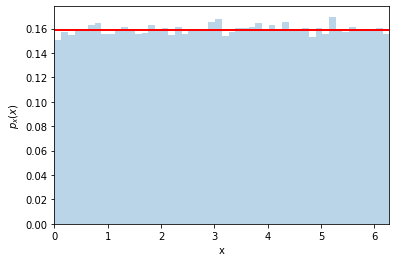Ok, now let’s make a histogram for $$y=\cos(x)$$

counts, y_bins, patches = plt.hist(y, bins=50, density=True, alpha=0.3)
plt.xlabel('y')
plt.ylabel('$p_y(y)$')

Text(0, 0.5, '$p_y(y)$')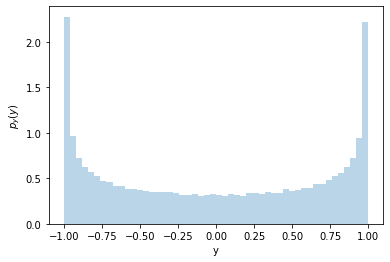It’s not uniform! Why is that? Let’s look at the $$x-y$$ relationship

# make a scatter of x,y
plt.scatter(x[:300],y[:300]) #just the first 300 points

xtest = .2
plt.plot((-1,xtest),(np.cos(xtest),np.cos(xtest)), c='r')
plt.plot((xtest,xtest),(-1.5,np.cos(xtest)), c='r')
xtest = xtest+.1
plt.plot((-1,xtest),(np.cos(xtest),np.cos(xtest)), c='r')
plt.plot((xtest,xtest),(-1.5,np.cos(xtest)), c='r')

xtest = 2*np.pi-xtest
plt.plot((-1,xtest),(np.cos(xtest),np.cos(xtest)), c='g')
plt.plot((xtest,xtest),(-1.5,np.cos(xtest)), c='g')
xtest = xtest+.1
plt.plot((-1,xtest),(np.cos(xtest),np.cos(xtest)), c='g')
plt.plot((xtest,xtest),(-1.5,np.cos(xtest)), c='g')

xtest = np.pi/2
plt.plot((-1,xtest),(np.cos(xtest),np.cos(xtest)), c='r')
plt.plot((xtest,xtest),(-1.5,np.cos(xtest)), c='r')
xtest = xtest+.1
plt.plot((-1,xtest),(np.cos(xtest),np.cos(xtest)), c='r')
plt.plot((xtest,xtest),(-1.5,np.cos(xtest)), c='r')

xtest = 2*np.pi-xtest
plt.plot((-1,xtest),(np.cos(xtest),np.cos(xtest)), c='g')
plt.plot((xtest,xtest),(-1.5,np.cos(xtest)), c='g')
xtest = xtest+.1
plt.plot((-1,xtest),(np.cos(xtest),np.cos(xtest)), c='g')
plt.plot((xtest,xtest),(-1.5,np.cos(xtest)), c='g')

plt.ylim(-1.5,1.5)
plt.xlim(-1,7)

(-1.0, 7.0)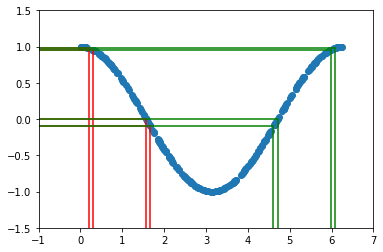The two sets of vertical lines are both separated by $$0.1$$. The probability $$P(a < x < b)$$ must equal the probability of $$P( cos(b) < y < cos(a) )$$. In this example there are two different values of $$x$$ that give the same $$y$$ (see green and red lines), so we need to take that into account. For now, let’s just focus on the first part of the curve with $$x<\pi$$.

So we can write (this is the important equation):

(6)$\begin{equation} \int_a^b p_x(x) dx = \int_{y_b}^{y_a} p_y(y) dy \end{equation}$

where $$y_a = \cos(a)$$ and $$y_b = \cos(b)$$.

and we can re-write the integral on the right by using a change of variables (pure calculus)

(7)$\begin{equation} \int_a^b p_x(x) dx = \int_{y_b}^{y_a} p_y(y) dy = \int_a^b p_y(y(x)) \left| \frac{dy}{dx}\right| dx \end{equation}$

notice that the limits of integration and integration variable are the same for the left and right sides of the equation, so the integrands must be the same too. Therefore:

(8)$\begin{equation} p_x(x) = p_y(y) \left| \frac{dy}{dx}\right| \end{equation}$

and equivalently

(9)$\begin{equation} p_y(y) = p_x(x) \,/ \,\left| \, {dy}/{dx}\, \right | \end{equation}$

The factor $$\left|\frac{dy}{dx} \right|$$ is called a Jacobian. When it is large it is stretching the probability in $$x$$ over a large range of $$y$$, so it makes sense that it is in the denominator.

plt.plot((0.,1), (0,.3))
plt.plot((0.,1), (0,0), lw=2)
plt.plot((1.,1), (0,.3))
plt.ylim(-.1,.4)
plt.xlim(-.1,1.6)
plt.text(0.5,0.2, '1', color='b')
plt.text(0.2,0.03, 'x', color='black')
plt.text(0.5,-0.05, 'y=cos(x)', color='g')
plt.text(1.02,0.1, '$\sin(x)=\sqrt{1-y^2}$', color='r')

Text(1.02, 0.1, '$\\sin(x)=\\sqrt{1-y^2}$')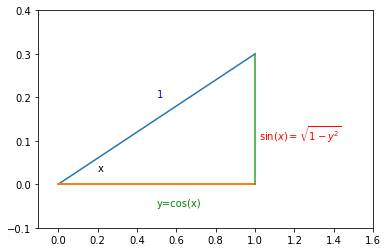In our case:

(10)$\begin{equation} \left|\frac{dy}{dx} \right| = \sin(x) \end{equation}$

Looking at the right-triangle above you can see $$\sin(x)=\sqrt{1-y^2}$$ and finally there will be an extra factor of 2 for $$p_y(y)$$ to take into account $$x>\pi$$. So we arrive at

(11)$\begin{equation} p_y(y) = 2 \times \frac{1}{2 \pi} \frac{1}{\sin(x)} = \frac{1}{\pi} \frac{1}{\sin(\arccos(y))} = \frac{1}{\pi} \frac{1}{\sqrt{1-y^2}} \end{equation}$

Notice that when $$y=\pm 1$$ the pdf is diverging. This is called a caustic and you see them in your coffee and rainbows!Let’s check our prediction

counts, y_bins, patches = plt.hist(y, bins=50, density=True, alpha=0.3)
pdf_y = (1./np.pi)/np.sqrt(1.-y_bins**2)
plt.plot(y_bins, pdf_y, c='r', lw=2)
plt.ylim(0,5)
plt.xlabel('y')
plt.ylabel('$p_y(y)$')

Text(0, 0.5, '$p_y(y)$')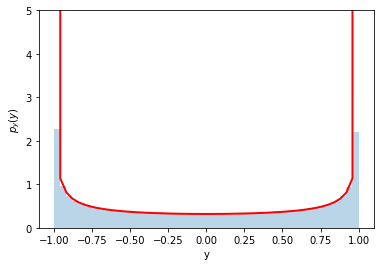Perfect!

## A trick using the cumulative distribution function (cdf) to generate random numbers¶

Let’s consider a different variable transformation now – it is a special one that we can use to our advantage.

(12)$\begin{equation} y(x) = \textrm{cdf}(x) = \int_{-\infty}^x p_x(x') dx' \end{equation}$

Here’s a plot of a distribution and cdf for a Gaussian.

(NOte: the axes are different for the pdf and the cdf http://matplotlib.org/examples/api/two_scales.html

from scipy.stats import norm

x_for_plot = np.linspace(-3,3, 30)
fig, ax1 = plt.subplots()

ax1.plot(x_for_plot, norm.pdf(x_for_plot), c='b')
ax1.set_ylabel('p(x)', color='b')
for tl in ax1.get_yticklabels():
tl.set_color('b')

ax2 = ax1.twinx()
ax2.plot(x_for_plot, norm.cdf(x_for_plot), c='r')
ax2.set_ylabel('cdf(x)', color='r')
for tl in ax2.get_yticklabels():
tl.set_color('r')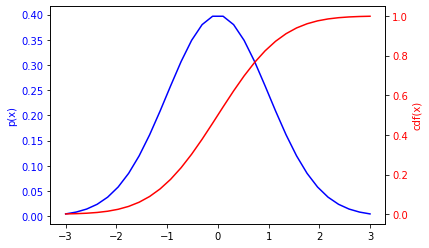Ok, so let’s use our result about how distributions transform under a change of variables to predict the distribution of $$y=cdf(x)$$. We need to calculate

(13)$\begin{equation} \frac{dy}{dx} = \frac{d}{dx} \int_{-\infty}^x p_x(x') dx' \end{equation}$

Just like particles and anti-particles, when derivatives meet anti-derivatives they annihilate. So $$\frac{dy}{dx} = p_x(x)$$, which shouldn’t be a surprise.. the slope of the cdf is the pdf.

So putting these together we find the distribution for $$y$$ is:

(14)$\begin{equation} p_y(y) = p_x(x) \, / \, \frac{dy}{dx} = p_x(x) /p_x(x) = 1 \end{equation}$

So it’s just a uniform distribution from $$[0,1]$$, which is perfect for random numbers.

We can turn this around and generate a uniformly random number between $$[0,1]$$, take the inverse of the cdf and we should have the distribution we want for $$x$$.

Let’s try it for a Gaussian. The inverse of the cdf for a Gaussian is called ppf

norm.ppf.__doc__

'\n        Percent point function (inverse of cdf) at q of the given RV.\n\n        Parameters\n        ----------\n        q : array_like\n            lower tail probability\n        arg1, arg2, arg3,... : array_like\n            The shape parameter(s) for the distribution (see docstring of the\n            instance object for more information)\n        loc : array_like, optional\n            location parameter (default=0)\n        scale : array_like, optional\n            scale parameter (default=1)\n\n        Returns\n        -------\n        x : array_like\n            quantile corresponding to the lower tail probability q.\n\n        '

#check it out
norm.cdf(0), norm.ppf(0.5)

(0.5, 0.0)


Ok, let’s use CDF trick to generate Normally-distributed (aka Gaussian-distributed) random numbers

rand_cdf = np.random.uniform(0,1,10000)
rand_norm = norm.ppf(rand_cdf)

_ = plt.hist(rand_norm, bins=30, density=True, alpha=0.3)
plt.xlabel('x')

Text(0.5, 0, 'x')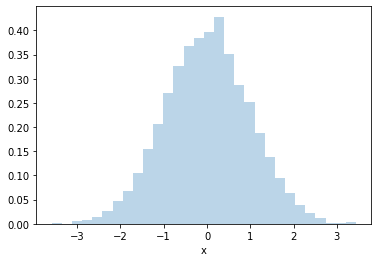Pros: The great thing about this technique is it is very efficient. You only generate one random number per random $$x$$.

Cons: the downside is you need to know how to compute the inverse cdf for $$p_x(x)$$ and that can be difficult. It works for a distribution like a Gaussian, but for some random distribution this might be even more computationally expensive than the accept/reject approach. This approach also doesn’t really work if your distribution is for more than one variable.

## Going full circle¶

Ok, let’s try it for our distribution of $$y=\cos(x)$$ above. We found

(15)$\begin{equation} p_y(y) = \frac{1}{\pi} \frac{1}{\sqrt{1-y^2}} \end{equation}$

So the CDF is (see Wolfram alpha for integral )

(16)$\begin{equation} cdf(y') = \int_{-1}^{y'} \frac{1}{\pi} \frac{1}{\sqrt{1-y^2}} = \frac{1}{\pi}\arcsin(y') + C \end{equation}$

and we know that for $$y=-1$$ the CDF must be 0, so the constant is $$1/2$$ and by looking at the plot or remembering some trig you know that it’s also $$cdf(y') = (1/\pi) \arccos(y')$$.

So to apply the trick, we need to generate uniformly random variables $$z$$ between 0 and 1, and then take the inverse of the cdf to get $$y$$. Ok, so what would that be:

(17)$\begin{equation} y = \textrm{cdf}^{-1}(z) = \cos(\pi z) \end{equation}$

Of course! that’s how we started in the first place, we started with a uniform $$x$$ in $$[0,2\pi]$$ and then defined $$y=\cos(x)$$. So we just worked backwards to get where we started. The only difference here is that we only evaluate the first half: $$\cos(x < \pi)$$